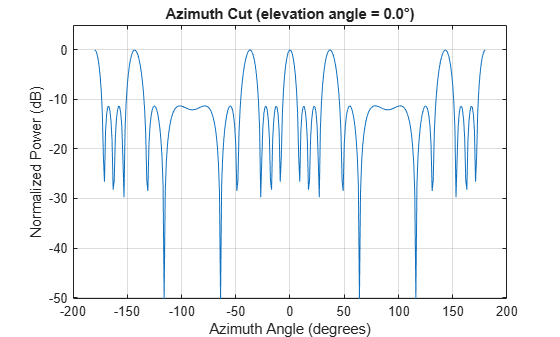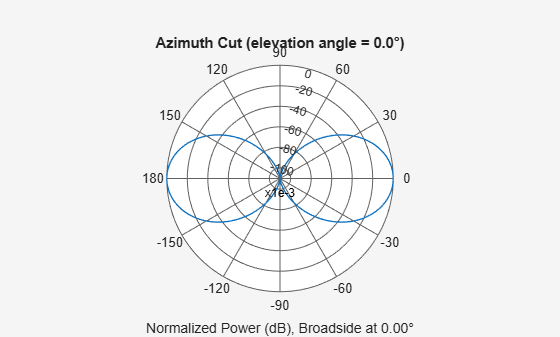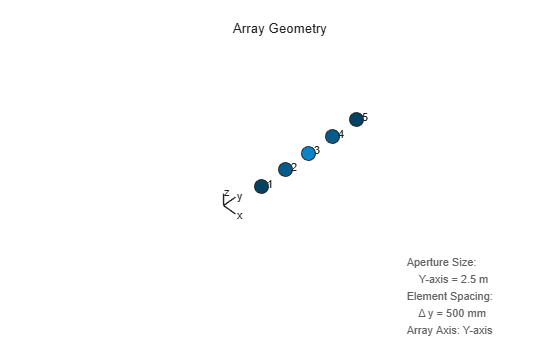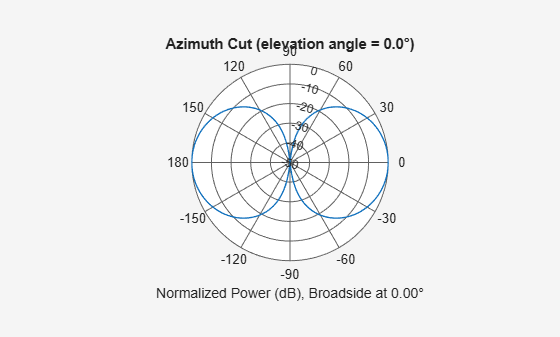phased.ULA

Uniform linear array

Description

The phased.ULA System object™ creates a uniform linear array (ULA).

To compute the response for each element in the array for specified directions:

1. Define and set up your uniform linear array. See Construction.

2. Call step to compute the response according to the properties of phased.ULA. The behavior of step is specific to each object in the toolbox.

Note

Starting in R2016b, instead of using the step method to perform the operation defined by the System object, you can call the object with arguments, as if it were a function. For example, y = step(obj,x) and y = obj(x) perform equivalent operations.

Construction

H = phased.ULA creates a uniform linear array (ULA) System object, H. The object models a ULA formed with identical sensor elements. The origin of the local coordinate system is the phase center of the array. The positive x-axis is the direction normal to the array, and the elements of the array are located along the y-axis.

H = phased.ULA(Name,Value) creates object, H, with each specified property Name set to the specified Value. You can specify additional name-value pair arguments in any order as (Name1,Value1,...,NameN,ValueN).

H = phased.ULA(N,D,Name,Value) creates a ULA object, H, with the NumElements property set to N, the ElementSpacing property set to D, and other specified property Names set to the specified Values. N and D are value-only arguments. When specifying a value-only argument, specify all preceding value-only arguments. You can specify name-value pair arguments in any order.

Properties

Element

Element of array

Specify the element of the sensor array as a handle. The element must be an element object in the phased package.

Default: Isotropic antenna element with default array properties

NumElements

Number of elements

An integer containing the number of elements in the array.

Default: 2

ElementSpacing

Element spacing

A scalar containing the spacing (in meters) between two adjacent elements in the array.

Default: 0.5

ArrayAxis

Array axis

Array axis, specified as one of 'x', 'y', or 'z'. ULA array elements are located along the selected coordinate system axis.

Element normal vectors are determined by the selected array axis

ArrayAxis Property ValueElement Normal Direction
'x'azimuth = 90°, elevation = 0° (y-axis)
'y'azimuth = 0°, elevation = 0° (x-axis)
'z'azimuth = 0°, elevation = 0° (x-axis)

Default: 'y'

Taper

Element tapering

Element tapering or weighting, specified as a complex-valued scalar, 1-by-N row vector, or N-by-1 column vector. In this vector, N represents the number of elements of the array. Tapers, also known as weights, are applied to each sensor element in the sensor array and modify both the amplitude and phase of the received data. If 'Taper' is a scalar, the same taper value is applied to all elements. If 'Taper' is a vector, each taper value is applied to the corresponding sensor element.

Default: 1

Methods

Specific to phased.ULA Object
beamwidth

Compute and display beamwidth of an array

collectPlaneWave

directivity

Directivity of uniform linear array

getElementNormal

Normal vector to array elements

getElementPosition

Positions of array elements

getNumElements

Number of elements in array

getTaper

Array element tapers

isPolarizationCapable

Polarization capability

pattern

Plot array pattern

patternAzimuth

Plot ULA array directivity or pattern versus azimuth

patternElevation

Plot ULA array directivity or pattern versus elevation

plotGratingLobeDiagram

Plot grating lobe diagram of array

plotResponse

Plot response pattern of array

step

Output responses of array elements

viewArray

View array geometry

Common to All System Objects
release

Allow System object property value changes

Examples

collapse all

Create a 4-element undersampled ULA and find the response of each element at boresight. Plot the array pattern at 1 GHz for azimuth angles between -180 and 180 degrees. The default element spacing is 0.5 meters.

array = phased.ULA('NumElements',4);
fc = 1e9;
ang = [0;0];
resp = array(fc,ang)
resp = 4×1

1
1
1
1

c = physconst('LightSpeed');
pattern(array,fc,-180:180,0,'PropagationSpeed',c,...
'CoordinateSystem','rectangular',...
'Type','powerdb','Normalize',true)Construct a 10-element uniform linear array of omnidirectional microphones spaced 3 cm apart. Then, plot the array pattern at 100 Hz.

mic = phased.OmnidirectionalMicrophoneElement(...
'FrequencyRange',[20 20e3]);
Nele = 10;
array = phased.ULA('NumElements',Nele,...
'ElementSpacing',3e-2,...
'Element',mic);
fc = 100;
ang = [0; 0];
resp = array(fc,ang);
c = 340;
pattern(array,fc,[-180:180],0,'PropagationSpeed',c,...
'CoordinateSystem','polar',...
'Type','powerdb',...
'Normalize',true);Build a tapered uniform line array of 5 short-dipole sensor elements. Because short dipoles support polarization, the array should as well. Verify that it supports polarization by looking at the output of the isPolarizationCapable method.

antenna = phased.ShortDipoleAntennaElement(...
'FrequencyRange',[100e6 1e9],'AxisDirection','Z');
array = phased.ULA('NumElements',5,'Element',antenna,...
'Taper',[.5,.7,1,.7,.5]);
isPolarizationCapable(array)
ans = logical
1

Then, draw the array using the viewArray method.

viewArray(array,'ShowTaper',true,'ShowIndex','All')Compute the horizontal and vertical responses.

fc = 150e6;
ang = ;
resp = array(fc,ang);

Display the horizontal polarization response.

resp.H
ans = 5×1

0
0
0
0
0

Display the vertical polarization response.

resp.V
ans = 5×1

-0.6124
-0.8573
-1.2247
-0.8573
-0.6124

Plot an azimuth cut of the vertical polarization response.

c = physconst('LightSpeed');
pattern(array,fc,[-180:180],0,...
'PropagationSpeed',c,...
'CoordinateSystem','polar',...
'Polarization','V',...
'Type','powerdb',...
'Normalize',true)References

 Brookner, E., ed. Radar Technology. Lexington, MA: LexBook, 1996.

 Van Trees, H. Optimum Array Processing. New York: Wiley-Interscience, 2002.

Extended Capabilities

Introduced in R2011a